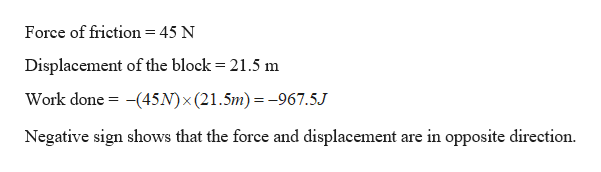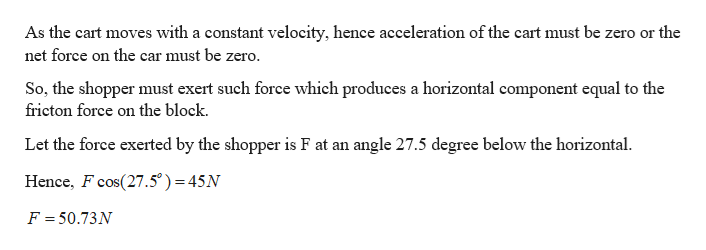# A shopper pushes a grocery cart for a distance 21.5 m at a constant speed on level ground, against a 45 N frictional force. He pushes in a direction 27.5° below the horizontal.a.) What is the work done on the cart by friction, in joules? b.) Find the magnitude of the force, in newtons, that the shopper exerts on the cart.c.) What is the total work done on the cart, in joules?

Question
12 views

A shopper pushes a grocery cart for a distance 21.5 m at a constant speed on level ground, against a 45 N frictional force. He pushes in a direction 27.5° below the horizontal.

a.) What is the work done on the cart by friction, in joules?

b.) Find the magnitude of the force, in newtons, that the shopper exerts on the cart.

c.) What is the total work done on the cart, in joules?

check_circle

Step 1

Given:

Displacement of the cart = 21.5 m

Friction force = 45 N

Direction of the force =  27.5° below the horizontal

Step 2

(a) Calculating the work done on the car by friction:help_outlineImage TranscriptioncloseForce of friction = 45 N Displacement of the block 21.5 m (45N) x (21.5m)=-967.5J Work done Negative sign shows that the force and displacement are in opposite direction fullscreen
Step 3

(b) Calculating the magnitude of force th...help_outlineImage TranscriptioncloseAs the cart moves with a constant velocity, hence acceleration of the cart must be zero or the net force on the car must be zero. So, the shopper must exert such force which produces a horizontal component equal to the fricton force on the block. Let the force exerted by the shopper is F at an angle 27.5 degree below the horizontal Hence, F cos(27.5°) 45N = F = 50.73N fullscreen

### Want to see the full answer?

See Solution

#### Want to see this answer and more?

Solutions are written by subject experts who are available 24/7. Questions are typically answered within 1 hour.*

See Solution
*Response times may vary by subject and question.
Tagged in

### Newtons Laws of Motion